Start typing, then use the up and down arrows to select an option from the list.Jules Bruno
322views
2
So let's take a look at the first two examples and see how we approach these types of questions. We say here when we have a solution where a gcN two minus gas, C N minus gas and a G positive gas and they have an equilibrium constant K equal to 1.8 times 10 to the negative 19. If the equilibrium concentrations of a gcN two minus and c n minus, are these numbers respectively? What is the equilibrium concentration of a G positive? So we ask ourselves do we use a nice chart or not here? I give you the equilibrium amounts of this compound which is 0.30. And this compound which is 0.10, you're missing only one equilibrium amount. If you're missing only one equilibrium amount, then you don't have to do a nice chart. So we're simply going to say in this case that K equals products. Overreact ints we say that products here would be C n minus squared times a G positive. And since we're gonna need some room guys, I'm gonna remove myself from the image. So we have more room to work with divided by AGCN two negative. So what we're gonna do now Is we're gonna plug in the numbers that we know we know what K is. It's 1.8 times 10 to the negative 19 CN negatively said was .10 and it's gonna be squared eight G positive is what we're looking for. So we're gonna have it as a variable divided by a gcN two minus which is 20.30. Okay, so this question becomes fairly easy. All we have to do now is isolate RAG positive. So we're gonna do first is multiply both sides by .030. Yeah. Yeah. These two multiply together to give us a new answer of 5.4 times 10 to the -21 And that equals .10 squared times a G positive. We just want a G positive. So we're gonna divide it out the 0.10 squared. So our final answer at the end for silver ion will be 5.4 times 10 to the negative 19 moller. So again, why didn't we have to use an ice chart? We didn't have to use a nice chart because we're missing only one person at equilibrium. When you're missing no one at equilibrium or you're missing only one variable at equilibrium. Then you don't need to do a nice chart. We only do a nice chart when more than when more than one concentration is missing at equilibrium.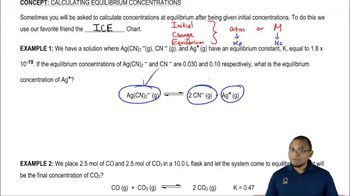02:29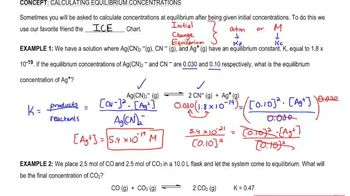03:19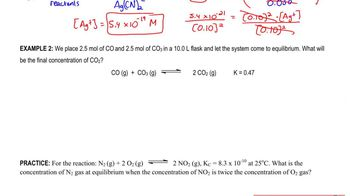07:40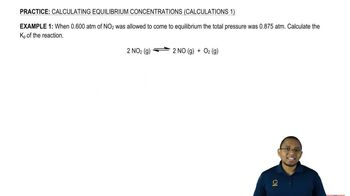05:56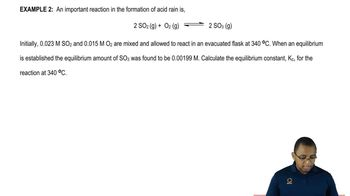06:13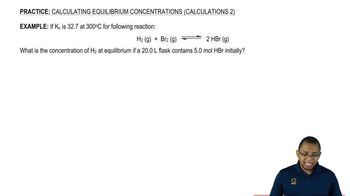07:56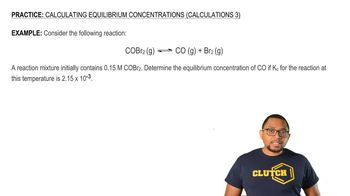07:19